## RS Aggarwal Solutions Class 10 Chapter 14 Height and Distance Ex 14a

These Solutions are part of RS Aggarwal Solutions Class 10. Here we have given RS Aggarwal Solutions Class 10 Chapter 14 Height and Distance Ex 14a.

Other Exercises

Question 1.
Solution:
Let AB be the tower standing on a level ground and O be the position of the observer. Then OA = 20 m and ∠OAB = 90° and ∠AOB = 60°Let AB = h meters
From the right ∆OAB, we haveQuestion 2.
Solution:
Let OB be the length of the string from the level of ground and O be the point of the observer, then, AB = 75m and ∠OAB = 90° and ∠AOB = 60°, let OB = l meters.
From the right ∆OAB, we have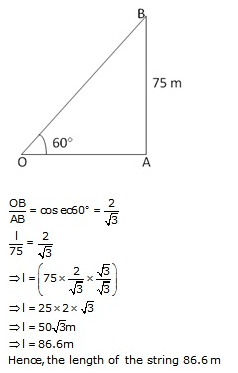Question 3.
Solution: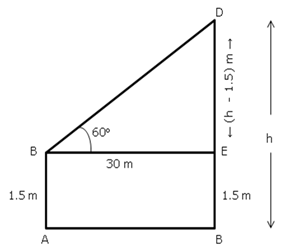Question 4.
Solution: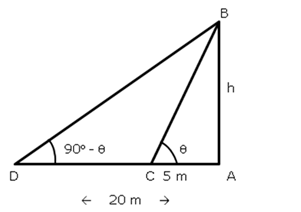Question 5.
Solution: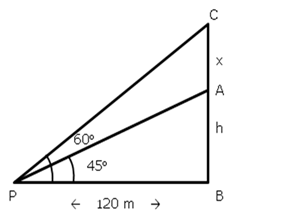Question 6.
Solution: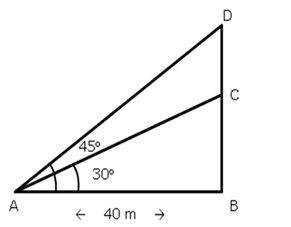Question 7.
Solution: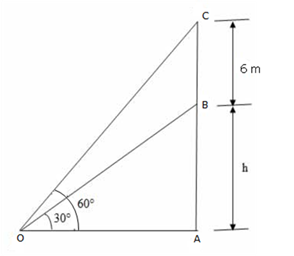Question 8.
Solution:
Let SP be the statue and PB be the pedestal. Angles of elevation of S and P are 60° and 45° respectively.
Further suppose AB = x m, PB = h mQuestion 9.
Solution:
Let AB be the unfinished tower and let AC be complete tower.
Let O be the point of observation. Then,
OA = 75 m
∠AOB = 30° and ∠AOC = 60°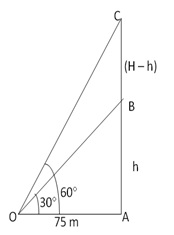Question 10.
Solution:
Let AB be the tower and BC be flagpole, Let O be the point of observation.
Then, OA = 9 m, ∠AOB = 30° and ∠AOC = 60°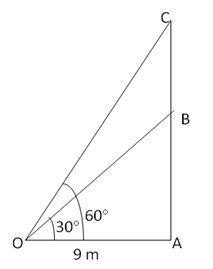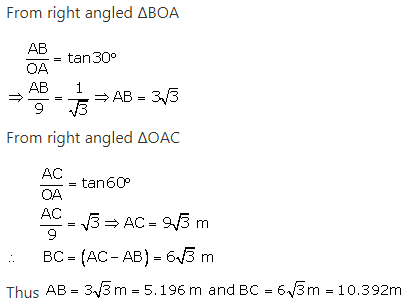Hence, height of the tower= 5.196 m and the height of the flagpole = 10.392 m.

Question 11.
Solution: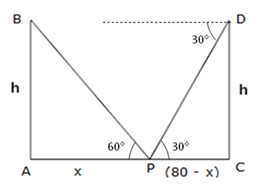Question 12.
Solution:Question 13.
Solution:Question 14.
Solution: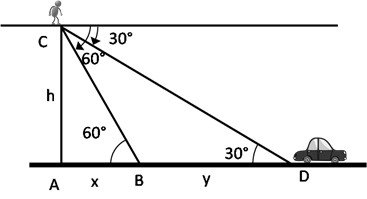Question 15.
Solution: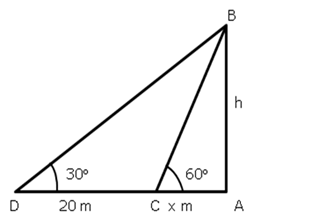Question 16.
Solution: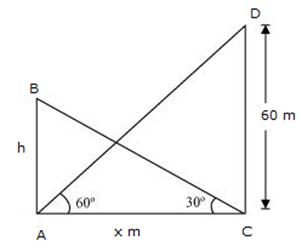Question 17.
Solution:
Let AB and CD be the first and second towers respectively.
Then, CD = 90 m and AC = 60 m.
Let DE be the horizontal line through D.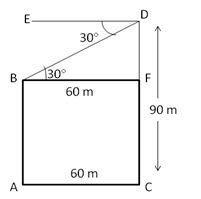Draw BF ⊥CD,
Then, BF = AC = 60 m
∠FBD = ∠EDB = 30°Question 18.
Solution:Question 19.
Solution: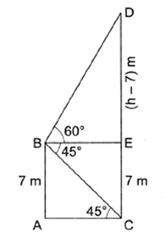Question 20.
Solution:Question 21.
Solution: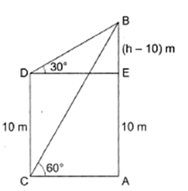Question 22.
Solution:Question 23.
Solution:
Let AB be the height of the deck and let CD be the cliff..
Let the man be at B, then, AB= 16 m
Let BE ⊥CD and AE ⊥ CD
Then, ∠EBD = 60 and ∠EBC = 30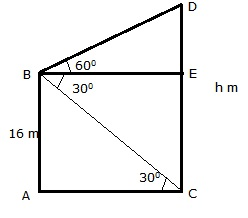CE = AB = 16m
Let CD = h meters
Then, ED = (h 16)m
From right ∆BED, we have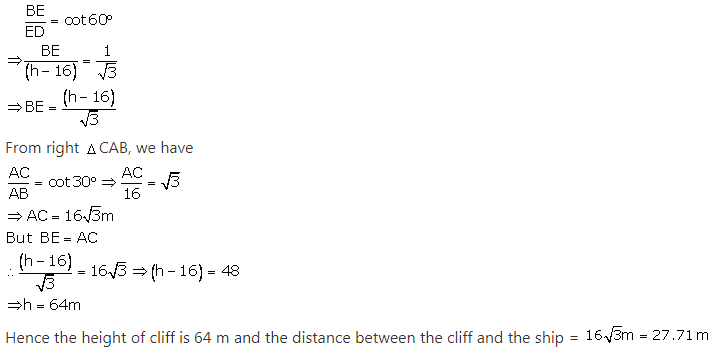Question 24.
Solution: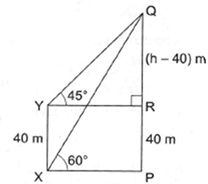Question 25.
Solution: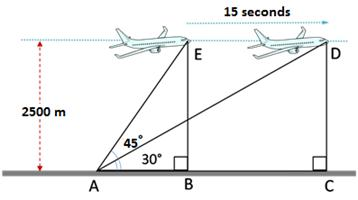Question 26.
Solution:
Let AB be the tower and let the angle of elevation of its top at C be 30°.
Let D be a point at a distance 150 m from C such that the angle of elevation of the top of tower at D is 60°.
Let h m be the height of the tower and AD = x m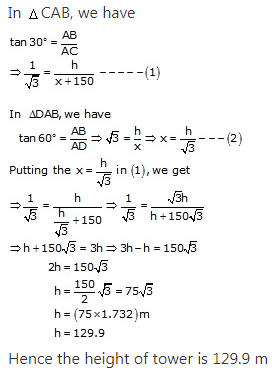Question 27.
Solution:
Let AB be the light house and let C and D be the positions of the ship.
Let AD =x, CD = y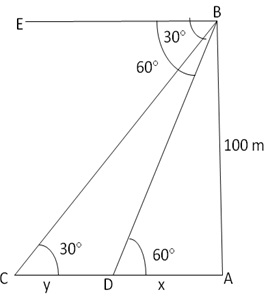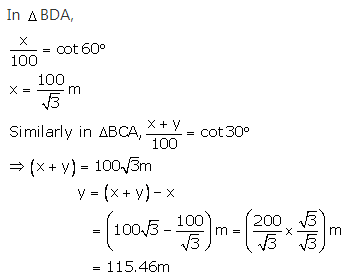The distance travelled by the ship during the period of observation = 115.46 m

Question 28.
Solution:Question 29.
Solution: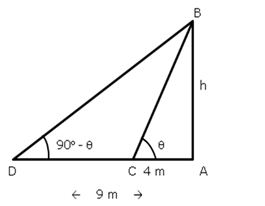Question 30.
Solution: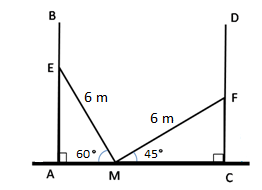Question 31.
Solution: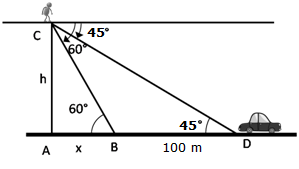Question 32.
Solution: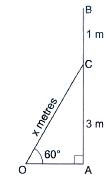Question 33.
Solution: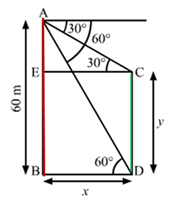Hope given RS Aggarwal Solutions Class 10 Chapter 14 Height and Distance Ex 14a are helpful to complete your math homework.

If you have any doubts, please comment below. Learn Insta try to provide online math tutoring for you.